Test: Three Phase Converters - 2

# Test: Three Phase Converters - 2

Test Description

## 20 Questions MCQ Test Topicwise Question Bank for Electrical Engineering | Test: Three Phase Converters - 2

Test: Three Phase Converters - 2 for Electrical Engineering (EE) 2023 is part of Topicwise Question Bank for Electrical Engineering preparation. The Test: Three Phase Converters - 2 questions and answers have been prepared according to the Electrical Engineering (EE) exam syllabus.The Test: Three Phase Converters - 2 MCQs are made for Electrical Engineering (EE) 2023 Exam. Find important definitions, questions, notes, meanings, examples, exercises, MCQs and online tests for Test: Three Phase Converters - 2 below.
Solutions of Test: Three Phase Converters - 2 questions in English are available as part of our Topicwise Question Bank for Electrical Engineering for Electrical Engineering (EE) & Test: Three Phase Converters - 2 solutions in Hindi for Topicwise Question Bank for Electrical Engineering course. Download more important topics, notes, lectures and mock test series for Electrical Engineering (EE) Exam by signing up for free. Attempt Test: Three Phase Converters - 2 | 20 questions in 20 minutes | Mock test for Electrical Engineering (EE) preparation | Free important questions MCQ to study Topicwise Question Bank for Electrical Engineering for Electrical Engineering (EE) Exam | Download free PDF with solutions
 1 Crore+ students have signed up on EduRev. Have you?
Test: Three Phase Converters - 2 - Question 1

### A three-phase full converter supplied from a 230 V source is working as a line commutated inverter. The load consists of RLE type with R = 5 Ω, E = 200 V and L = 1 mH. A continues current of 10 A is flowing through the load, find the value of the firing angle delay.

Detailed Solution for Test: Three Phase Converters - 2 - Question 1

Vo = 200 – 10×5 = 150V as the circuit is operating as an inverter Vo = -150V
Now, Vo = (3Vml/π) cos α
α = cos-1(-150π/3√2×230) = 118.88°.

Test: Three Phase Converters - 2 - Question 2

### A three-phase full converter is driving a DC motor. If a continues current of Im amperes is flowing through the motor load, then find the rms value of supply current drawn by the converter to drive the motor.

Detailed Solution for Test: Three Phase Converters - 2 - Question 2

The RMS value of the supply current IS over π radians would be
(IS)2 = (1/π) x (Im)2 x (2π/3) = Im√2/√3.

Test: Three Phase Converters - 2 - Question 3

### Name the below given circuit.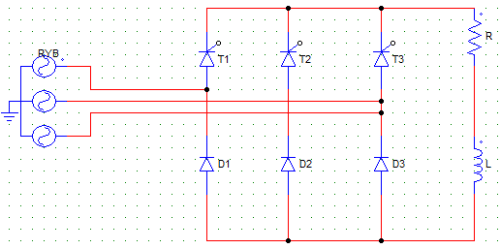Detailed Solution for Test: Three Phase Converters - 2 - Question 3

It uses 3 SCRs and 3 diodes, hence it is a semi-converter. Option (b) and (d) make no sense, because there can be no full controlled semi-converter.

Test: Three Phase Converters - 2 - Question 4

In the below given circuit, each SCR and diode conduct for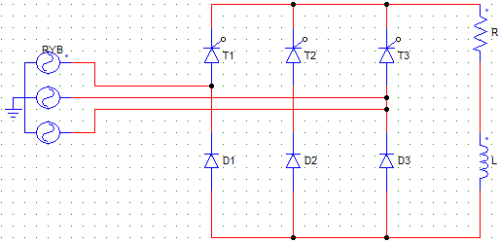Detailed Solution for Test: Three Phase Converters - 2 - Question 4

At any given time, one SCR and one diode is conducting, each conduct for 120° per cycle.

Test: Three Phase Converters - 2 - Question 5

In the below given circuit, __ and __ conduct along with T2.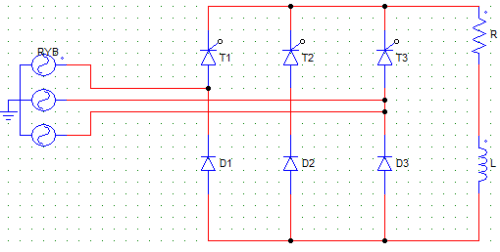Detailed Solution for Test: Three Phase Converters - 2 - Question 5

When one SCR conducts, a diode conducts along with it at a time to provide the path of current flow. . For example, if T2 starts conducting at 90° it will conduct till 90+120 = 210°. But while T2 is conducting, half of the time i.e. from 90 to 150 D1 is conducting and another half of the time D3 is conducting. T2 and D2 cannot conduct together as it will cause a short circuit. Hence, T2-D1 conduct for 60° and then T2-D3 conduct for another 60°.

Test: Three Phase Converters - 2 - Question 6

In a three-phase semi-converter, at a time one SCR and one diode conduct simultaneously. With SCR T1 conducting which diode(s) is most likely to conduct along with T1?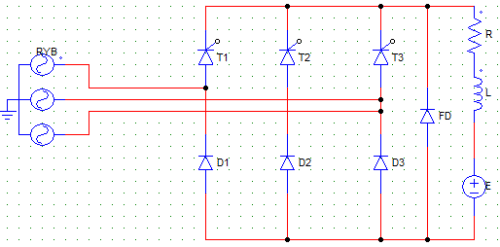Detailed Solution for Test: Three Phase Converters - 2 - Question 6

T1 and D1 together will cause a S.C. D2 or D3 any of these two can conduct along with T1 depending on which phase voltage is currently active RB or RY.

Test: Three Phase Converters - 2 - Question 7

What is the value of voltage at the output terminal when the freewheeling diode (FD) is conducting?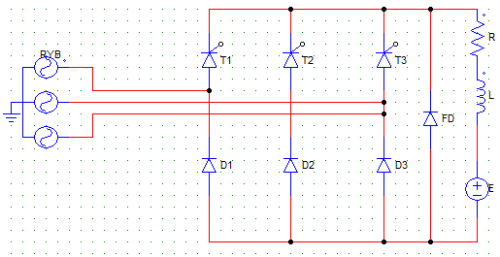Detailed Solution for Test: Three Phase Converters - 2 - Question 7

When FD is conducting it will short circuit the load terminal resulting in zero voltage. It won’t be E because the terminals are shorted. It can be E when none of the devices are conducting (This can happen only when α > 120°).

Test: Three Phase Converters - 2 - Question 8

A 3-phase full converter feeds power to an R load of 10 Ω. For a firing angle delay of 30° the load takes 5 kW. An inductor of large value is also connected to the load to make the current ripple free. Find the value of per phase input voltage.

Detailed Solution for Test: Three Phase Converters - 2 - Question 8

Ior = Vo/R = (3Vml/Rπ) cos α
P = 5 kW = Ior2 x R = [(3Vml/π) cos α]2 x 1/R] Therefore, Vs (line) = √50000 x (π/√2 x 3 x cos30) = 191.22 V
Vs (phase) = 191/√3 V.

Test: Three Phase Converters - 2 - Question 9

A three-phase semi-converter circuit is given a supply of 400 V. It produces at the output terminals an average voltage of 381 V. Find the rectification efficiency of the converter circuit.

Detailed Solution for Test: Three Phase Converters - 2 - Question 9

Rectification efficiency = Pdc/Pac
Pdc = Vo x Io . . . (both average values of output current and voltage)
Pac = Vrms x Irms . . . (both rms values of input current and voltage)
For a semi-converter Irms = Io
Therefore, Rectification efficiency = 381/400 = 95.25 %.

Test: Three Phase Converters - 2 - Question 10

In a 3-phase semi-converter, for firing angle less than 60° the freewheeling diode conducts for

Detailed Solution for Test: Three Phase Converters - 2 - Question 10

In case of a semi-converter operating with α < 60°, FD does not comes into play, as the voltage never falls to zero and gives no chance for the inductor to discharge.

Test: Three Phase Converters - 2 - Question 11

In a 3-phase full wave converter, if V is the maximum value of line voltage at the input, then each SCR is subjected to a peak negative voltage of

Detailed Solution for Test: Three Phase Converters - 2 - Question 11

PIV = √3 Vml in case of a semi-converter.

Test: Three Phase Converters - 2 - Question 12

In a 3-phase semi-converter, firing angle is less than 60°, as such each SCR and diode conduct respectively for __________ (in degrees)

Detailed Solution for Test: Three Phase Converters - 2 - Question 12

Each will conduct for 120° per cycle whatever the firing angle is.

Test: Three Phase Converters - 2 - Question 13

The effect of source inductance on the performance of a 3-phase controlled converter is to

Detailed Solution for Test: Three Phase Converters - 2 - Question 13

It reduces the average value of the output voltage by introducing a overlap delay μ.

Test: Three Phase Converters - 2 - Question 14

Which of the following converter circuits would require a neutral point?

Detailed Solution for Test: Three Phase Converters - 2 - Question 14

Half wave converter would require delta-star transformer, the secondary winding star connection requires a neutral point.

Test: Three Phase Converters - 2 - Question 15

The range of firing angle for a 3-phase, 3-pulse converter feeding a resistive load is __________ (in degrees).

Detailed Solution for Test: Three Phase Converters - 2 - Question 15

Firing angle for a 3-phase, 3-pulse converter feeding a resistive load is 0 to 150 degrees.

Test: Three Phase Converters - 2 - Question 16

A 3-phase bridge converter is given a three-phase supply in the phase sequence R-Y-B. Let the neutral to R phase voltage be Vm sinωt. The first SCR (connected to R phase) is fired at an angle of 15°. What is the maximum value at the output terminals at this instant?

Detailed Solution for Test: Three Phase Converters - 2 - Question 16

In case of a 3 phase bridge converter, the maximum value of voltage at the output terminal is always 1.5 Vm.

Test: Three Phase Converters - 2 - Question 17

The PIV experienced by each SCRs in M-3 converter is __________ times that in a 3-phase full converter having the same output voltage.

Detailed Solution for Test: Three Phase Converters - 2 - Question 17

In case of M-3 type of connection, the devices have to handle more peak inverse voltage (PIV) than the 3-phase full converter which has 6 SCRs.

Test: Three Phase Converters - 2 - Question 18

Each SCR of a 3-phase 6-pulse converter conducts for

Detailed Solution for Test: Three Phase Converters - 2 - Question 18

A 3-phase 6-pulse converter is nothing but the 3-pulse full controlled converter using 6 devices each conducting for 120°.

Test: Three Phase Converters - 2 - Question 19

A 3-phase full converter has an average output voltage of 365 V for zero degree firing and resistive load. For a firing angle of 90 degree, the output voltage would be

Detailed Solution for Test: Three Phase Converters - 2 - Question 19

Cos 90 = 0.

Test: Three Phase Converters - 2 - Question 20

Semi-converters are

Detailed Solution for Test: Three Phase Converters - 2 - Question 20

Semi-converters are single quadrant converters, because the voltage and current can only be both positive due to the diodes connected.

## Topicwise Question Bank for Electrical Engineering

207 tests
 Use Code STAYHOME200 and get INR 200 additional OFF Use Coupon Code
Information about Test: Three Phase Converters - 2 Page
In this test you can find the Exam questions for Test: Three Phase Converters - 2 solved & explained in the simplest way possible. Besides giving Questions and answers for Test: Three Phase Converters - 2, EduRev gives you an ample number of Online tests for practice

207 tests